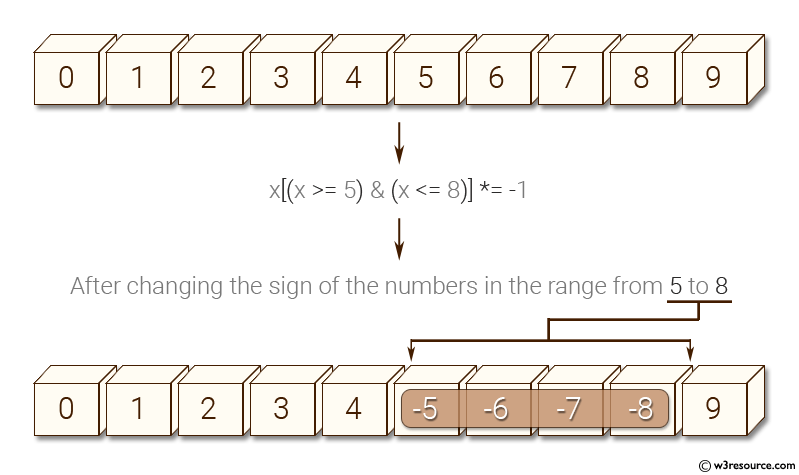﻿ NumPy: Create a vector with values ​​from 0 to 20 and change the sign of the numbers in the range from 9 to 15 - w3resource# NumPy: Create a vector with values ​​from 0 to 20 and change the sign of the numbers in the range from 9 to 15

## NumPy: Basic Exercise-22 with Solution

Write a NumPy program to create a vector with values ​​from 0 to 20 and change the sign of the numbers in the range from 9 to 15.

Sample Solution :

Python Code :

``````import numpy as np
x = np.arange(20)
print("Original vector:")
print(x)
print("After changing the sign of the numbers in the range from 9 to 15:")
x[(x >= 9) & (x <= 15)] *= -1
print(x)
``````

Sample Output:

```Original vector:
[ 0  1  2  3  4  5  6  7  8  9 10 11 12 13 14 15 16 17 18 19]
After changing the sign of the numbers in the range from 9 to 15:
[  0   1   2   3   4   5   6   7   8  -9 -10 -11 -12 -13 -14 -15  16  17
18  19]
```

Pictorial Presentation:Python Code Editor:

Have another way to solve this solution? Contribute your code (and comments) through Disqus.

What is the difficulty level of this exercise?

Test your Python skills with w3resource's quiz

﻿

## Python: Tips of the Day

Merges two or more lists into a list of lists, combining elements from each of the input lists based on their positions

Example:

```def tips_merge(*args, fill_value=None):
max_length = max([len(lst) for lst in args])
result = []
for i in range(max_length):
result.append([
args[k][i] if i < len(args[k])
else fill_value for k in range(len(args))
])
return result
print(tips_merge(['x', 'y'], [1, 2], [True, False]))
print(tips_merge(['x'], [1, 2], [True, False]))
print(tips_merge(['x'], [1, 2], [True, False], fill_value = '_'))
```

Output:

```[['x', 1, True], ['y', 2, False]]
[['x', 1, True], [None, 2, False]]
[['x', 1, True], ['_', 2, False]]
```